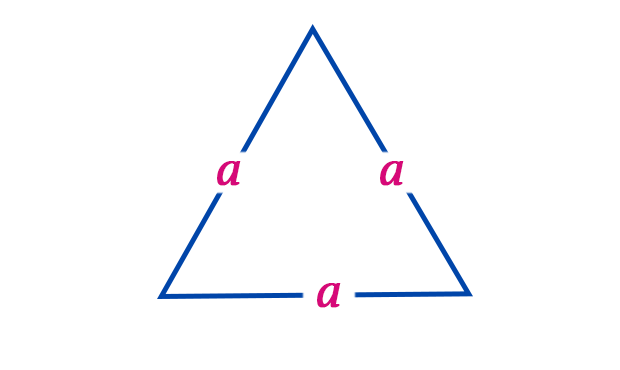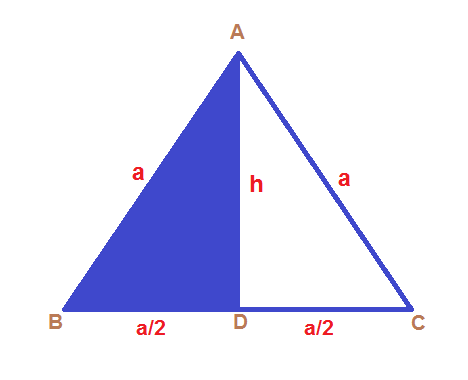# Area of An Equilateral Triangle• An equilateral triangle is a triangle in which all 3 sides are equal.
• Equilateral triangles are also equiangular, which means, all three internal angles are also equal to each other and the only value possible is 60° each.
• The area of the equilateral triangle is basically the amount of space occupied by an equilateral triangle.
• The area of a triangle is measured in unit2.
• In an equilateral triangle, the median, angle bisector, and perpendicular are all the same and can be simply termed as the perpendicular bisector due to congruence conditions.
• A triangle is equilateral if and only if any 3 of the smaller triangles have either the same perimeter or the same inradius.
• A triangle is equilateral if and only if the circumcenters of any three of the smaller triangles have the same distance from the centroid.

Area of Equilateral Triangle Formula:  A = √3/4a2

where “a” denoted the sides of an Equilateral Triangle

Proof:In the figure above, the sides of an equilateral triangle are equal to “a” units.

We know that the area of Triangle is given by;

A = 1/2×base×height

To find the height, consider Triangle ABC,
Applying Pythagoras Theorem we know,

a2=h2+(a/2)2

h2=a2a2/4

h2=3a2/4

h=√3a/2

Thus, we can calculate area by a basic equation,
A = 1/2×b×h=1/2×a×√3a/2 Therefore, A = =√3a2/4unit2

### Let’s work out a few examples:-

Example 1: Find the area of an equilateral triangle whose side is 7 cm?

Solution:

Given,

Side of the equilateral triangle = a = 7 cm

Area of an equilateral triangle =  √3/4 a2

√3/4×72 cm2

√3/4×49 cm2

= 21.21762 cm2

Example 2: Find the area of an equilateral triangle whose side is 28 cm?

Solution:

Given,

Side of the equilateral triangle (a) = 28 cm

We know, Area of an equilateral triangle = √3/4 a2

√3/4×282 cm2

√3/4×784 cm2

= 339.48196 cm2